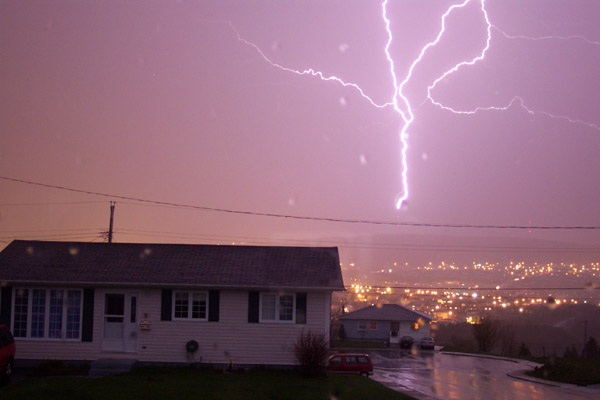# Difference Between Charge and Current

## Main Difference – Charge vs. Current

Electricity operates thanks to a property of particles called charge. The main difference between charge and current is that charge refers to a fundamental property of a particle while current refers to the rate of flow of charge.

## What is Charge

Charge is a fundamental property of particles that allows them to interact with each other via electromagnetic forces. During these interactions, charge is always conserved. Electric charges could be either positive or negative. A positive charge refers to the charge carried by a proton while a negative charge refers to the charge carried by an electron. Like charges repel each other while unlike charges attract. The force of attraction or repulsion$F$ between two particles having charges$q_1$ and$q_2$ which are separated by a distance$r$ is given by Coulomb’s law:$F=k\frac{q_1q_2}{r^2}$

where$k$ is a constant, with$k=$ 8.99×109 m F-1.

Even though everyday objects are made of protons and electrons, they are often not considered to be charged because the positive and the negative charges cancel each other out, leaving no net charge. Sometimes, if two objects are rubbed against each other electrons can be transferred from one object to the other, leaving one object with excess electrons and the other with excess protons. Then the objects are charged. If the charged objects are not allowed to come into contact with a conductor, electrons cannot travel to/from the object easily and so the charge stays on for a considerable length of time. This type of situation where excess charges are accumulated on a body is often referred to as static electricity. Lightning happens when static electricity builds up in clouds, and then suddenly discharge.Lightning happens when the excess charges in clouds discharge.

Charge is measured in units of coulombs (C). An electron has a charge of about -1.6×10-19 C.

## What is Current

In electricity, current refers to the rate of flow of charge. A current$I$ is expressed in terms of charges$Q$ as:$I=\frac{\mathrm dQ}{\mathrm dt}$

Currents are measured in units of amperes (A). A current of 1 A refers to a flow of charge where 1 C of charges flow past a point every second. For electrical circuits, the law of conservation of charge extends into a law of conservation of current. At every junction in a circuit, the sum of currents entering the junction must be equal to the sum of currents leaving that junction (this is also known as Kirchoff’s first law).

The currents that we encounter every day are created by electrons travelling through wires in response to a potential difference. Currents could be direct (DC) or alternating (AC). Often, people mistakenly assume that electrons need  to travel from the place where the circuit is switched on, to a device in order to make it work. This is not true. When a circuit is switched on, electrons all over the wire begin to move in response almost simultaneously. Typically, electron speeds (called “drift speeds“) through a wire are actually very slow, of the order of about a centimetre per minute. In AC currents, electrons largely stay where they are, and instead they only move back and forth periodically.

## Difference Between Charge and Current

Charge is a fundamental property of particles that allows them to interact with electromagnetic forces. It is measured in coulombs.

Current is a rate of flow of charge. It is measured in amperes (A).

Image Courtesy

“Lightning Crashes” by Ian (Own work) [CC BY-SA 2.0], via flickr